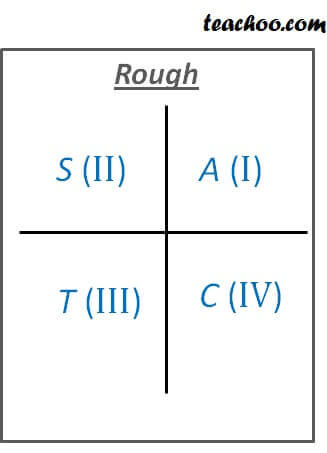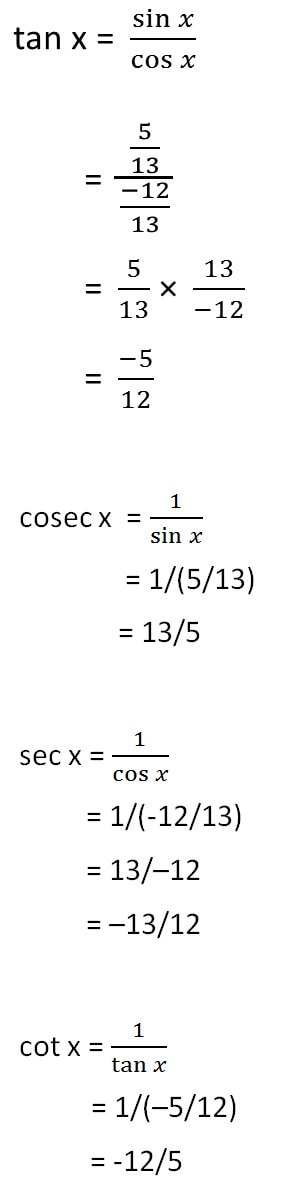Finding Value of trignometric functions, given other functions

Chapter 3 Class 11 Trigonometric Functions
Concept wise

Let’s take an example

#### If cos x = –12/13 lies in second quadrant, find value of other five trigonometric functions

Since x is in 2nd Quadrant,tan and cos will be negative

But sin will be positive

Given cos x = –12/13

We know that

sin 2 x  +  cos 2 x = 1

sin 2 x  +  (-12/13) 2 = 1

sin 2 x  +  144/169 = 1

sin 2 x = 1 – 144/169

sin 2 x = (169 – 144)/169

sin 2 x = 25/169

sin x = ±√(25/169)

sin x = ±√25 / √169

sin x = ± 5/13

Since, x is in 2nd Quadrant

sin x is positive

∴ sin x = 5/13Learn in your speed, with individual attention - Teachoo Maths 1-on-1 Class

### Transcript

Let s take an example If cos x = 12/13 lies in second quadrant, find value of other five trigonometric functions Since x is in 2nd Quadrant, tan and cos will be negative But sin will be positive Given cos x = 12/13 We know that sin2 x + cos2 x = 1 sin2 x + (-12/13)2 = 1 sin2 x + 144/169 = 1 sin2 x = 1 144/169 sin2 x = (169 144)/169 sin2 x = 25/169 sin x = (25/169) sin x = 25 / 169 sin x = 5/13 Since, x is in 2nd Quadrant sin x is positive sin x = 5/13